Vectors and conics

This free course is available to start right now. Review the full course description and key learning outcomes and create an account and enrol if you want a free statement of participation.

Free course

Vectors and conics

We saw above that the vector 2v can be regarded as the vector v ‘followed by’ the vector v; we can also quite naturally describe this vector as being the ‘sum’, v + v, of the vector with itself.

Analogously, if p is the vector 2 cm E and q is the vector 3 cm NE, we can think of the ‘sum’ p + q of the vectors as follows. Starting from a given point, O say, draw the vector p; starting from its finishing point, draw the vector q. Then if the final point reached is S, p + q is the vector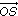.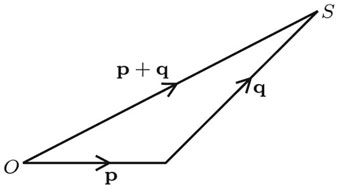Triangle Law for addition of vectors

The sum p + q of two vectors p and q is obtained as follows.

1. Starting at any point, draw the vector p.

2. Starting from the finishing point of the vector p, draw the vector q.

Then the sum p + q is the vector from the starting point of p to the finishing point of q.

There is an equivalent way of visualising the sum of vectors geometrically. Instead of following p with q to find the third side of a triangle, as above, we obtain exactly the same vector if we draw p and q with the same starting point, complete the parallelogram of which these are adjacent sides, and take the diagonal of the parallelogram from the common starting point (see below).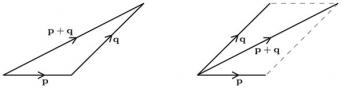Parallelogram Law for addition of vectors

The sum p + q of two vectors p and q is obtained as follows.

1. Starting at the same point, draw the vectors p and q.

2. Complete the parallelogram of which these are adjacent sides.

Then the sum p + q is the vector from the starting point of p and q to the opposite corner of the parallelogram.

The order in which we add vectors does not matter: the sum q + p is precisely the same vector as the sum p + q. It turns out also that the sums (p + q) + r and p + (q + r) are equal. Thus addition of vectors is commutative and associative. (We omit the proof.)

Finally, we can define subtraction of vectors in terms of addition and scalar multiplication. For example, if p and q are the vectors 3.8 cm E and 2.4 cm NE, respectively, then −q is the vector 2.4 cm SW, and we define the difference pq to be the vector obtained by drawing first p and then −q.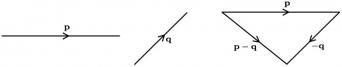Definition

The difference, pq, of two vectors p and q is pq = p + (−q).

In general, qp does not equal pq; in fact, as you would expect, qp = −(pq).

Since the vector −q has the same magnitude as q but the opposite direction, we can draw pq by using either of the two constructions which we use for adding vectors.

Example 21

For the vectors p and q shown below, sketch p + q, pq and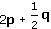.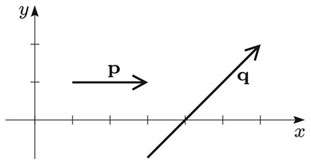First we sketch the vectors p, q, −q, 2p and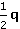.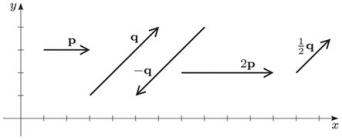We use the Triangle Law for the addition of vectors to sketch p + q, pq and.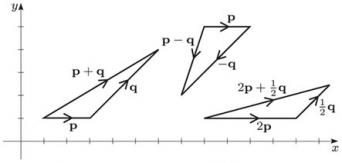M208_1